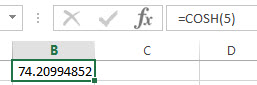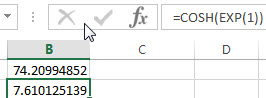# Excel COSH Function

This post will guide you how to use Excel COSH function with syntax and examples in Microsoft excel.

### Description

The Excel COSH function returns the hyperbolic cosine of a given angle.

The COSH function is a build-in function in Microsoft Excel and it is categorized as a Math and Trigonometry Function.

The COSH function is available in Excel 2016, Excel 2013, Excel 2010, Excel 2007, Excel 2003, Excel XP, Excel 2000, Excel 2011 for Mac.

### Syntax

The syntax of the COSH function is as below:

`= COSH(number)`

Where the COSH function argument is:

• number –This is a required argument. Any real number that you want to calculate the hyperbolic cosine.

### Excel COSH Function Examples

The below examples will show you how to use Excel COSH Function to calculate the hyperbolic cosine of a supplied number.

1# get the hyperbolic cosine of 5, enter the following formula in Cell B1.

=COSH(5)2# get the hyperbolic of the base of the natural logarithm, enter the following formula in Cell B2.

=COSH(EXP(1))### Related Functions

• Excel COS function
The Excel COS function returns the cosine of a given angle. The syntax of the COS function is as below:= COS(number)…
• Excel SIN function
The Excel TANH function returns the hyperbolic tangent of a given number.The syntax of the TANH function is as below:=TANH (number)…
• Excel ACOS function
The Excel ACOS function returns the arccosine value of a number.The ACOS function is a build-in function in Microsoft Excel and it is categorized as a Math and Trigonometry Function.The syntax of the ACOS function is as below:= ACOS(number)…
• Excel ACOSH function
The Excel ACOSH function returns the inverse hyperbolic cosine of a number.The syntax of the ACOSH function is as below:= ACOSH (number)…
• Excel EXP function
The Excel EXP function returns e raised to the power of a number.  The constant e is equal to 2.71828182845904, and it is the base of the natural logarithm. The syntax of the EXP function is as below:= EXP (number)…